# How to use Square Root in Excel

Feb 01, 2020 • edited Feb 02, 2020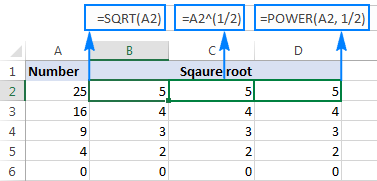A common engineering spreadsheet problem is solved by this quick tutorial on how to input square root.

The tutorial shows how to do square root in Excel as well as how to calculate Nth root of any value.

The square root of a number is a value that, when multiplied by itself, gives the number. The SQRT function in Excel returns the square root of a number.

1. First, to square a number, multiply the number by itself. For example, 4 * 4 = 16 or 4^2 = 16.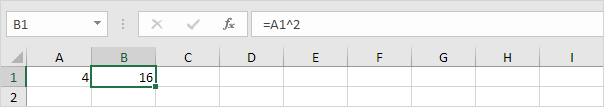Note: to insert a caret ^ symbol, press SHIFT + 6.

2. The square root of 16 is 4.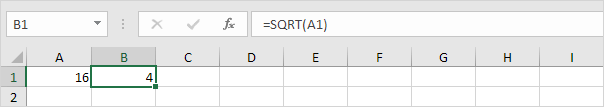3. Instead of using the SQRT function, you could also use an exponent of 1/2. Don't forget the parentheses.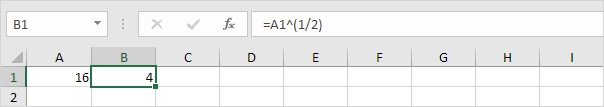4. If a number is negative, the SQRT function returns the #NUM! error.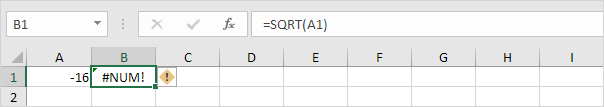5. You can use the ABS function to remove the minus sign (-) from a negative number.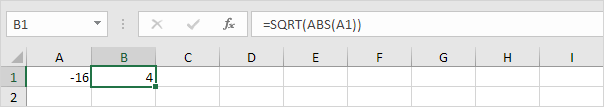Excel has no built-in function to calculate the nth root of a number. To calculate the nth root of a number, simply raise that number to the power of 1/n.

6. For example, 5 * 5 * 5 or 5^3 is 5 raised to the third power.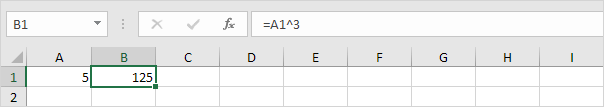7. The cube root of 125 is 5.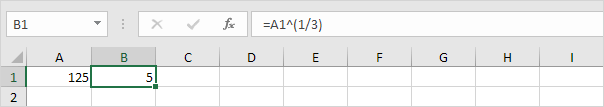8. For example, 2 * 2 * 2 * 2 * 2 * 2 or 2^6 is 2 raised to the sixth power.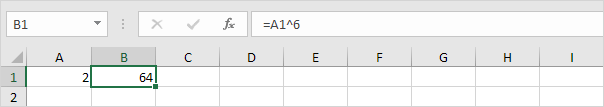9. The sixth root of 64 is 2.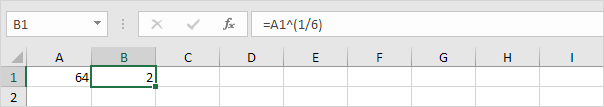#How To#Tutorial#Formulas#Functions

How to Calculating percentages with Formulas in Excel

How To Division Formula in Excel